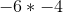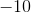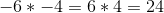Example Questions

← Previous 1

Example Question #1 : How To Subtract Negative Numbers

What is 1 + (–1) – (–3) + 4 ?

1

9

3

6

7

7

Explanation:

You simplify the expression to be 1 – 1 + 3 + 4 = 7

Example Question #2 : Negative Numbers

Solve the following equation for: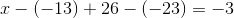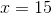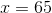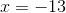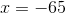Explanation:

To begin, we need to recall how to subtract negative numbers. Remember, when subtracting a negative number, the two negatives cancel out, creating a positive.

So, this:Becomes this: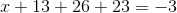We can combine like terms on the left to get: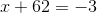Then, we can subtract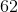from both sides in order to getby itself: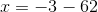In this case, we are subtracting from a negative number, which is just like adding two negative numbers, or subtracting from a positive number. The result will be more negative, because we will be moving further to the left on the number line.Example Question #1 : Describe Situations In Which Opposite Quantities Combine To Make 0: Ccss.Math.Content.7.Ns.A.1a

Compute the following: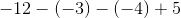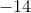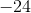Explanation:

Convert all the double signs to a single sign before solving. Remember, two minus (negative) signs combine to form a plus (positive) sign, and a plus (positive) sign and a minus (negative) sign combine to form a minus (negative) sign.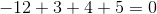Example Question #1 : How To Multiply Negative Numbers

If a = –2 and b = –3, then evaluate a3 + b2

8

1

17

9

5

1

Explanation:

When multiplying negative numbers, we get a negative answer if there are an odd number of negative numbers being multiplied.  We get a positive answer if there are an even number of negative numbers being multiplied.

a3 + b2 becomes (–2)3 + (–3)2 which equals –8 + 9 = 1

Example Question #1 : Negative Numbers

Evaluate:

–3 * –7

10

4

21

–10

–21

21

Explanation:

Multiplying a negative number and another negative number makes the product positive.

Example Question #6 : Negative Numbers

Evaluate.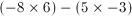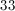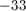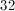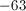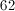Explanation:Multiplying a negative and a positive number creates a negative product: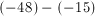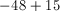Example Question #7 : Negative Numbers

Solve.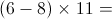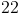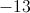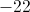Explanation: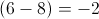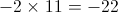A negative number multiplied by a positive number will always be negative.

Example Question #1 : How To Multiply Negative Numbers

Evaluate 3x3 + x2 if x = 2

28

14

22

20

20

Explanation:

When multiplying a negative number an odd number of times, the answer is negative.  When multiplying a negative number an even number of times, the answer is positive.  Order of operations also applies:  Parentheses, Exponents, Multiplication and Division, Addition and Subtraction, from left to right.  A mnemonic to remember the order of operations is “Please excuse my dear Aunt Sally.”

3(2)3 + (2)2

= 3(8) + (4)

= 24 + 4

= 20

Example Question #1 : How To Multiply Negative Numbers

Simplify the following expression: (–4)(2)(–1)(–3)

–16

12

24

–24

–24

Explanation:

First, we multiply –4 and 2. A negative and a positive number multiplied together give us a negative number, so (–4)(2) = –8. A negative times a negative is a positive so (–8)(–1) = 8. (8)(–3) = –24.

Example Question #10 : Negative Numbers

Evaluate the following: